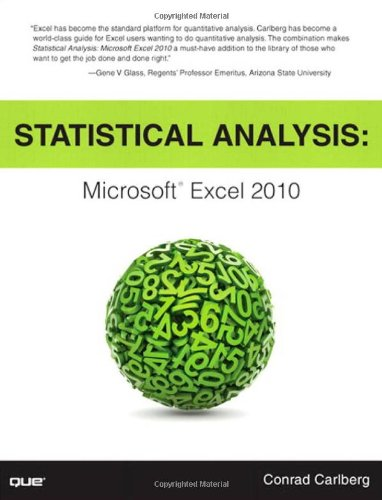# Statistical Analysis: Microsoft Excel 2010

Rating: 3.8 out of 5 49 reviews
• Length: 412 pages
• Edition: 1
• Publisher:
• Publication Date: 2011-05-02
• ISBN-10: 0789747200
• ISBN-13: 9780789747204

## Book Description

Top Excel guru Conrad Carlberg shows how to use Excel 2010 to perform the core statistical tasks every business professional, student, and researcher should master. Using real-world examples, Carlberg helps you choose the right technique for each problem and get the most out of Excel’s statistical features, including its new consistency functions. Along the way, you discover the most effective ways to use correlation and regression and analysis of variance and covariance. You see how to use Excel to test statistical hypotheses using the normal, binomial, t and F distributions.

Becoming an expert with Excel statistics has never been easier! You’ll find crystal-clear instructions, insider insights, and complete step-by-step projects—all complemented by an extensive set of web-based resources.

• Master Excel’s most useful descriptive and inferential statistical tools
• Tell the truth with statistics, and recognize when others don’t
• Accurately summarize sets of values
• View how values cluster and disperse
• Infer a population’s characteristics from a sample’s frequency distribution
• Explore correlation and regression to learn how variables move in tandem
• Understand Excel’s new consistency functions
• Test differences between two means using z tests, t tests, and Excel’s
• Use ANOVA and ANCOVA to test differences between more than two means
• Explore statistical power by manipulating mean differences, standard errors, directionality, and alpha

There is an Excel workbook for each chapter, and each worksheet is keyed to one of the book’s figures. You’ll also find additional material, such as a chart that demonstrates how statistical power shifts as you manipulate sample size, mean differences, alpha and directionality. To access these free files, please visit http://www.quepublishing.com/title/0789747200 and click the Downloads Tab.

``````1 About Variables and Values
2 How Values Cluster Together
3 Variability: How Values Disperse
4 How Variables Move Jointly: Correlation
5 How Variables Classify Jointly: Contingency Tables
6 Telling the Truth with Statistics
7 Using Excel with the Normal Distribution
8 Testing Differences Between Means: The Basics
9 Testing Differences Between Means: Further Issues
10 Testing Differences Between Means: The Analysis of Variance
11 Analysis of Variance: Further Issues
12 Multiple Regression Analysis and Effect Coding: The Basics
13 Multiple Regression Analysis: Further Issues
14 Analysis of Covariance: The Basics
15 Analysis of Covariance: Further Issues``````